## Multiple Reduction targets and Stop conditions

Disclaimer: The code in this post is written using version 9.1 of Simplygon. Should you encounter this post running a different version, some of the API calls might differ. However, the core concepts should still remain valid.

### Overview

Running reduction with multiple Reduction targets and Stop conditions is a flexible way to run the same reduction settings on a batch of assets of different sizes and complexity, while targeting both quality and performance goals.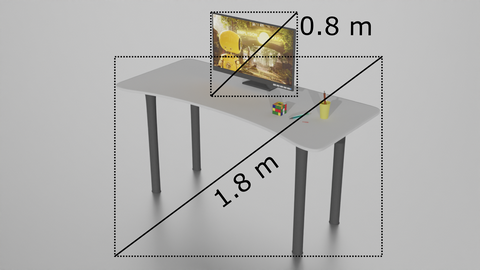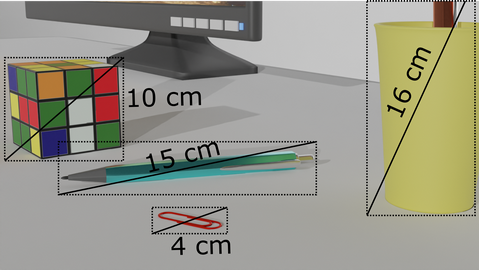### Reduction targets

There are four reduction targets, two that are quantity/performance budget based: Triangle Ratio and Triangle count and two that are quality based: Maximum deviation and On screen size. If you only specify either Triangle ratio or Triangle count, the reducer is instructed to run until a certain threshold of triangles have been removed, but you won't know what the resulting quality will be. Conversely, if you only set Max deviation or On screen size, you will guarantee a certain quality, but you might not reduce anything at all, or too much.

When you first start using Simplygon you'll probably use a single reduction target. It's not uncommon to make a cascaded LOD chain looking like this:

• Quantity based target
• LOD1: 50% triangles
• LOD2: 25% triangles
• LOD3: 12% triangles

That says little about the quality of the LODs however. To address that concern, you can set a quality based target that specifies something about how different the LODs are allowed to be from their originals:

• Quality based target
• LOD1: Look good at 5 meters from the camera (meaning the LOD switch distance is 5 meters, check out the guide on switch distance at Deviation and on screen size )
• LOD2: Look good at 10 meters from the camera
• LOD3: Look good at 20 meters from the camera

But if you run with these settings, you might ruin your triangle budget. If the model is an entire skyscraper and it should look good from a distance of 5 meters - well, it would require lots of triangles.

If you combine targets however, you can custom tailer a target with both the performance and the quality that you're aiming for.

• Combined quantity and quality targets
• LOD1: No more than 50% triangles allowed, but if quality isn't sacrificed, then keep reducing until a switch distance of 5 meters
• LOD2: 50% of LOD1's triangle count, but if quality isn't sacrificed, then keep reducing until a switch distance of 10 meters
• LOD2: 50% of LOD2's triangle count, but if quality isn't sacrificed, then keep reducing until a switch distance of 20 meters

If you run something like this. You'll be able to guarentee aspects about both the quality and the quantity of the LODs. For example with these settings, if the model being reduced is huge and complex, then the deciding factor will probably be the hard triangle limits 50%, 25%, 12% just like the first example. If a model is very small in comparison to the switch distance however, like a golf ball - then you'd probably be able to reduce a whole lot more than 50% triangles without sacrificing any perceivable quality from 5 meters away. You might end up with something like 20%, 10%, 5% in that case depending on the number of original triangles.

### Stop conditions

There are two stop conditions. Any is the conservative option. It instructs the reducer to only continue until the first target has been fulfilled. All is the aggressive option, it will run until all the criterias are met. For any object being reduced the four targets can be boiled down to two targets based on the geometric size and triangle count of the object.

Let's look at an instance where a mesh has 1k triangles, a diameter of 1 meter and the reduction targets are as follows: Triangle ratio: 0.5, Triangle count: 900, Max deviation: 0.1 m and On screen size: 20. The two quantity based targets can both be expressed as an exact triangle count target when we know the input model's triangle count; An explicit one from the actual Triangle count target setting, and an implict one from the Triangle ratio target setting.

Triangle ratio: 0.5Triangle count: 0.5 x 1000 triangles = 500 triangles.

The Stop condition: Any means that we want to terminate as soon as a target is met, so in this case we choose Triangle count: 900 (because 900 > 500).

For the quality based targets we can similarily convert the On screen size to a specific Max deviation value because we know the size of the model.

On screen size: 20Max deviation: 1 meter / 20 px = 0.05 meters.

As the mesh gets reduced, the deviation increases and since the Max deviation: 0.1 m target will be achieved first we can scrap the deviation from the On screen size.

To simplify:

• StopCondition: Any
• actual_triangle_count_target = max( explicit_triangle_count_target, implicit_triangle_count_target )
• actual_max_deviation_target = min( explicit_max_deviation_target, implicit_max_deviation_target )
• StopCondition: All
• actual_triangle_count_target = min( explicit_triangle_count_target, implicit_triangle_count_target )
• actual_max_deviation_target = max( explicit_max_deviation_target, implicit_max_deviation_target )

Where:
implicit_triangle_count_target = triangle_ratio_target x geometry_triangle_count
implicit_max_deviation_target = geometry_diameter / on_screen_size

### Example 1

Using this knowledge we can target a specific quality while enforcing a strict performance budget:

Stop condition: All
On screen size: 300 px
Triangle count: 10k

Result: The models should look good when being viewed at from the Switch distance but not at the expense of having more than 10k triangles. The Switch distance is determined by the Max deviation or On screen size and is computed per the method described in the documentation page: Deviation and on screen size .

### Example 2

Or we can target a specific performance budget while enforcing a certain assured quality:

Stop condition: Any
Triangle count: 5k
Triangle ratio: 0.1
Max deviation: 1 m

Result: The models reduce down to 5k triangles or to 10% of their original (when the original triangle count exceeds 50k) as long as the quality does not deteriorate to the point where the LOD moves more than a whole meter from the original.

### Example 3 (StopCondition: Any)

If you have a room full of scale diverse assets where some are larger: walls, doors, desk, chair, and some smaller: pens, paper clips, papers, cables - you'd probably want the larger assets to retain more triangles after reduction than the paper clips. This can be achieved by using the Max deviation reduction target and setting it to some small distance unit like 1 cm for instance. This means that an object being reduced will now be allowed to deviate 1 cm from the original. The door's indents and curve of the handle will lose details but the shape of the door will be very similar. The paper clip however, will probably be unrecognizable or close to it. But by using a combination of Reduction Targets you can still have 1 cm be primarily what's driving the reductions, but you can also make sure no asset is reduced past a certain triangle count, triangle ratio or an On Screen Size that marks a quality limit that shouldn't be reduced beyond.

The reduction of the large items in the room will primarily be driven by the Max deviation due to the nature of their size and complexity - but for the odd few smaller assets, they won't be turned into unrecognizable shapes.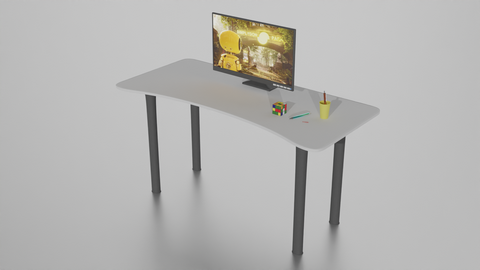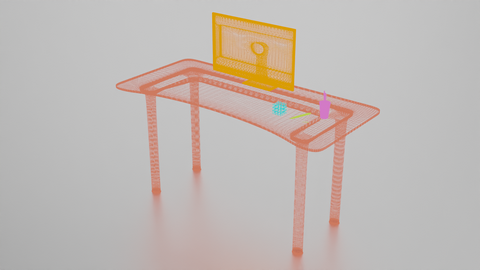Original scene WireframeDiameter of larger objects Diameter of smaller objects

Processing with these targets:

• Quantity based:
• Triangle ratio: 0.0 - Wants to decimate all the way to zero triangles
• Triangle count: 40 - Assures it never goes below 40 triangles
• Quality based:
• Max Deviation: 0.01 m = 1 cm - Assures the optimized object won't deviate more than 1 cm from the original model
• On Screen Size: 20 pixels - Makes sure the resulting geometry has an acceptable quality when occupying 20 pixels across on the screen

Now, to be able to interpret what happens we look at each asset being processed in order of descending size.

### Desk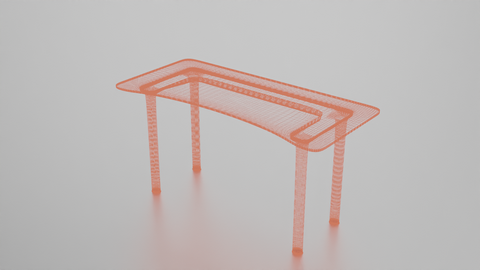Desk Desk wireframe

The Desk is the largest asset in the scene. It has a diameter of 1.8 meters and a 34k triangle count . Let's look at how far each reduction target means to decimate it.

• Targets
• Triangle ratio: 0.0 - Wants to decimate to zero triangles
• Triangle count: 40 - wants to decimate to 40 triangles
• Max deviation 0.01 - Wants to decimate to the point where it differs 1 cm from the original
• On screen size: 20 px - The size of the object means this target strives to reduce until the LOD differs (1.8m / 20px) = 0.090m = 9 cm from the original

Because the Stop condition is set to Any, the first met target will end the decimation. So it boils down to either Max(0, 40)=40 triangles, or Min(0.01, 0.09)=0.01 meters deviation (Keep in mind, smaller deviation is higher quality).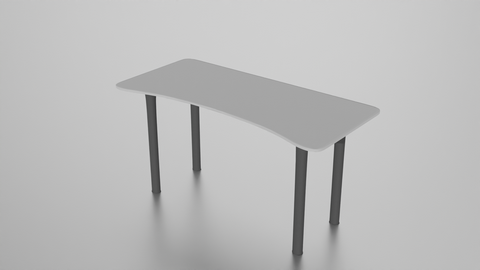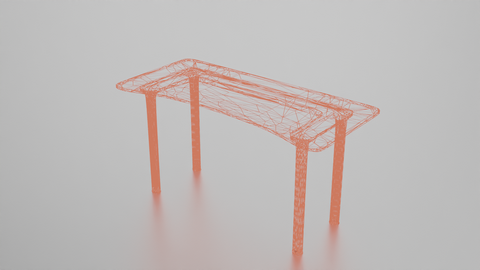Desk LOD Desk LOD wireframe

The LOD looks very much like the original model. There is hardly any perceivable difference when viewing from a moderate distance. The reduction target that was first met was Max deviation. This means the LOD was as far reduced as possible without sacrificing the intended quality target. It is reasonable to expect that assets larger than this asset and of comparable geometric complexity would also run until the max deviation target was met.

### MonitorMonitor Monitor wireframe

The Monitor is the second largest asset.

• Targets
• Triangle ratio: 0.0 - Wants to decimate to zero triangles
• Triangle count: 40 - wants to decimate to 40 triangles
• Max deviation 0.01 - Wants to decimate to the point where it differs 1 cm from the original
• On screen size: 20 px - The size of the object means this target strives to reduce until the LOD differs (0.8m / 20px) = 0.038m = 3.8 cm from the original

Once again, it boils down to either Max(0, 40)=40 triangles, or Min(0.01, 0.038)=0.01 meters deviation.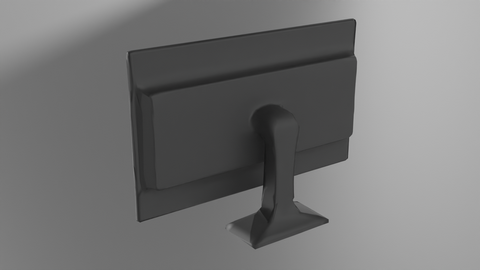Monitor LOD Monitor LOD wireframe

And just like the desk, because of the large size of the object in relation with the quality targets, the reduction target that was first met was Max deviation. We have however more closely zoomed in to make the object fill the screen and the difference between the original and reduced objects are starting to show.

### Cup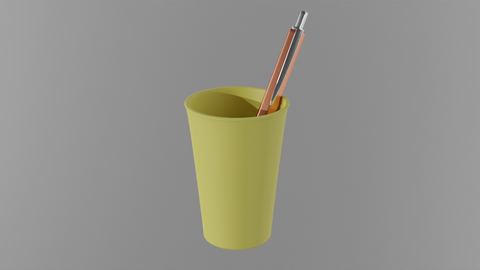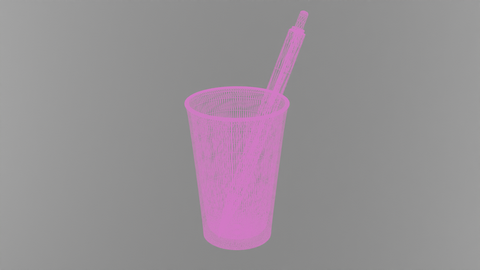Cup Cup wireframe
• Max deviation 0.01 - Wants to decimate to the point where it differs 1 cm from the original
• On screen size: 20 px - The size of the object means this target strives to reduce until the LOD differs (0.17m / 20px) = 0.0083m = 0.83 cm from the original

The Cup model is so small in relation to the On screen size that if we want to guarantee that the LOD is still looking acceptable when occupying 20 pixels on screen, we cannot let it deviate more than a mere 8.3 mm. This is the first object so far where our explicitly set Max deviation value is actually more coarse than the implicit one from On screen size.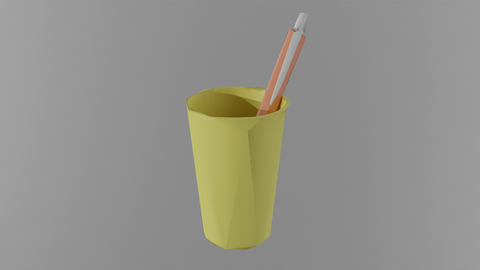Cup LOD Cup LOD wireframe

The reduction ran until the quality target from On screen size was met. The quality difference between Original and LOD is very much perceivable from this point.

### Pen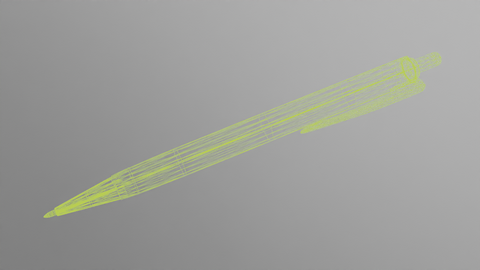Pen Pen wireframe
• Max deviation 0.01 - Wants to decimate to the point where it differs 1 cm from the original
• On screen size: 20 px - The size of the object means this target strives to reduce until the LOD differs (0.15m / 20px) = 0.0075m = 0.75 cm from the original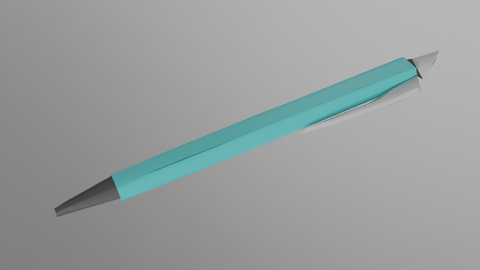Pen LOD Pen LOD wireframe

The reduction ran until the quality target from On screen size was met.

### Rubik's cube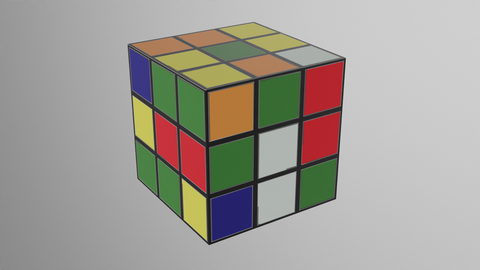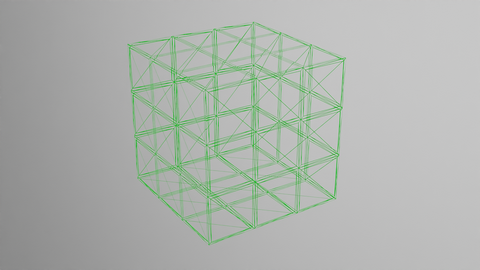Rubik's cube Rubik's cube wireframe

The Rubik's cube is the only object where quality isn't very affected by reducing triangles. The silhouette can be preserved very well down to about 12 triangles (6 sides x 2 triangles). That is why the first achieved Reduction target is quantity based and not quality based.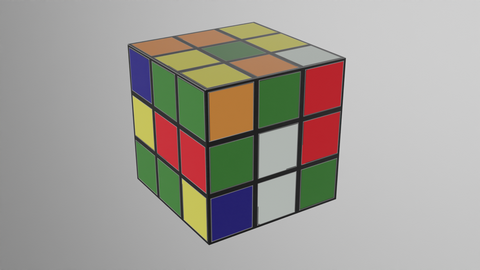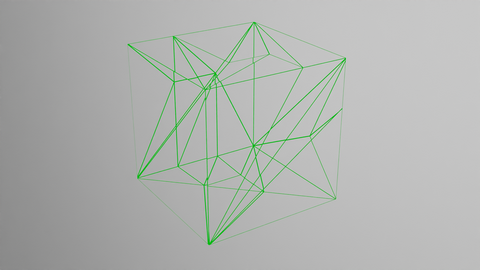Rubik's cubeLOD Rubik's cube LOD wireframe

The reduction ran until the quality target from Triangle count was met. The LOD doesn't look much different from the original.

### Paperclip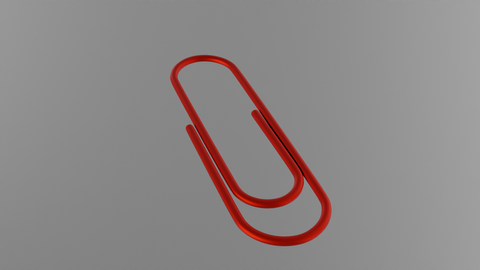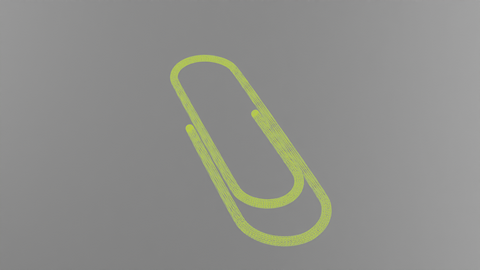Paperclip cube Paperclip wireframe
• Max deviation 0.01 - Wants to decimate to the point where it differs 1 cm from the original
• On screen size: 20 px - The size of the object means this target strives to reduce until the LOD differs (0.036 m / 20px) = 0.0018 = 0.18 cm from the original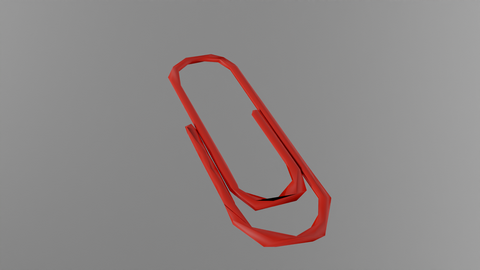Paperclip LOD Paperclip LOD wireframe

The reduction ran until the quality target from On screen size was met. The LOD looks very different from the original when viewed up close.

### Results

Desk Monitor Cup Pen Rubik's cube Paper clip
Diameter 1.8 m 0.8 m 0.16 m 0.15 m 0.097 m 0.036 m
Original triangle count 34,048 41,800 15,536 2,736 1,404 5,824
Resulting max deviation 0.01 m 0.01 m 0.0083 m 0.0075 m 0.0028 m 0.0017 m
Resulting on screen size 179 px 76 px 20 px 20 px 35 px 20 px
Resulting triangle count 5,794 1,522 1,242 261 40 278
Resulting triangle ratio 17% 3.6% 8.0% 9.5% 2.8% 4.8%
Deciding target - Max deviation - - Max deviation - - On screen size - - On screen size - - Triangle count - - On screen size -

From the table above we see that the two largest objects: Desk and Monitor ran until the desired quality of 1 cm deviation was achieved. However, the Cup, Pen and Paper clip were so small that the reducer had to stop when they would no longer have been able to look acceptable when taking up 20 pixels across on the screen. The outlier was the Rubik's cube with its simple geometry that could be reduced to the point where its triangle count matched the Triangle count target.

### Example 4 (StopCondition: All)

While Stop condition: Any makes sure you don't go beyond a certain point, Stop condition: All guarantees that all the targets are met. This can be particularily useful to ensure that a limit based on a performance quota is kept. Much like the example above you'd be able to target a specific quality using Max deviation but at the same time you can make sure that no reduced object has more than a selected number of triangles remaining.

The following scene is reduced with these targets:

Stop condition: All
Triangle count: 3,500 - Assures that there are at most 3,500 triangles
Max deviation: 1 meter - We know that we will be viewing the objects from a distance, so we don't care about details smaller than a meter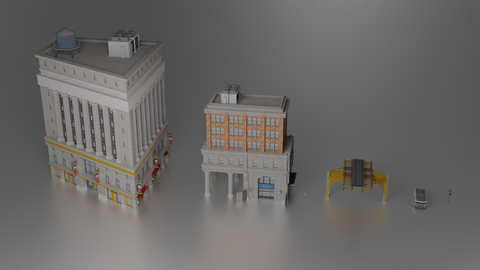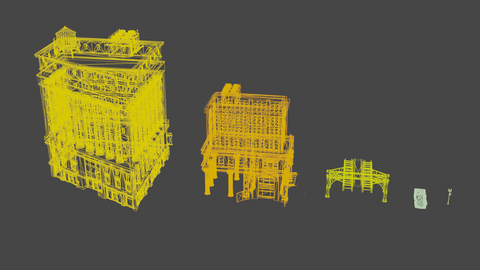Original scene Wireframe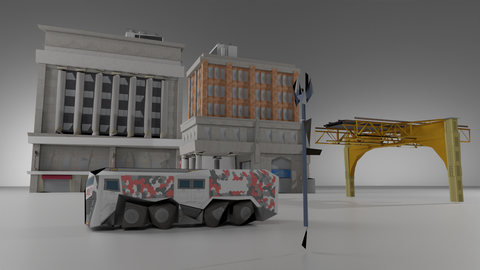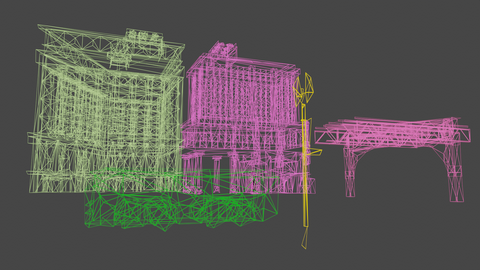LOD scene LOD Wireframe

#### Results

Large building Small building Tracks Vehicle Street light
Diameter 82 m 52 m 24 m 11 m 6 m
Original triangle count 34,779 21,138 2,544 6,704 2,578
Resulting max deviation 1.70 m 1.08 m 1.0 m 1.0 m 1.0 m
Resulting on screen size 48 px 48 px 25 px 11 px 6 px
Resulting triangle count 3,500 3,500 1,154 312 33
Resulting triangle ratio 10 % 17 % 45 % 4.7 % 1.3 %
Deciding target - Triangle count - - Triangle count - - Max deviation - - Max deviation - - Max deviation -

From the table above we see that the only two assets that actually ran past the quality target were the two largest objects; the buildings. The resulting Max deviation for the Large building became 1.70 meters after the strict triangle budget had been enforced. The Small building ran just past the quality target until it deviated 1.08 meters. The three smaller objects were reduced much further however, down to a minimum of 33 triangles for the street light which corresponds to 1.3% of its original triangle count. From the picture of the LODs the deteriorated quality of the Street light is really obvious up close but the resulting On screen size of the Street light is 6 pixels according to the table above (given its small size in relation to the maximum deviation). When viewed from the designed distances, all object look relatively OK.

### Code

The following code sets the Reduction targets and Stop condition in the Reduction settings.

`````` bool useTriangleRatio = true;
bool useTriangleCount = true;
bool useMaxDeviation = true;
bool useOnScreenSize = true;

// Selects which targets should be considered when reducing
reductionSettings->SetReductionTargets( EStopCondition::Any, useTriangleRatio, useTriangleCount, useMaxDeviation, useOnScreenSize );
reductionSettings->SetReductionTargetTriangleRatio( 0.0f );
reductionSettings->SetReductionTargetTriangleCount( 40 );
reductionSettings->SetReductionTargetMaxDeviation( 0.01f );
reductionSettings->SetReductionTargetOnScreenSize( 20 );
``````

After a reduction is complete you can fetch the Resulting deviation value from the spScene like this:

``````real ExtractMaxDeviation( spScene lodScene )
{
// Check that we got a MaxDeviation
spValueArray va = lodScene->GetCustomField( "MaxDeviation" );
if( va.IsNull() || va->GetItemCount() == 0 ){
printf( "Error: Expected MaxDeviation field with one item\n" );
}
spRealArray ra = spRealArray::SafeCast( va );
return ra->GetItem( 0 );
}
``````
⇐ Back to blog post list